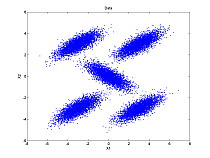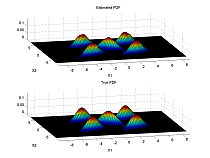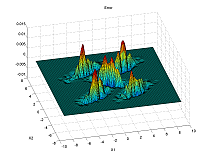## Biometrics Northwest LLC

Home

Services

Projects

Disclaimer

### Project: Nonparametric Probability Density Estimation

A MATLAB toolbox 'bsspdfest' implementing nonparametric probability function estimation using normalized B-splines was developed. The toolbox implements nonparametric probability function estimation procedures for one or more dimensions using a B-spline series for one-dimensional data and a tensor product B-spline series for multi-dimensional data. The toolbox takes advantage of the direct addressing of MATLAB arrays up to three dimensions and various vectorization approaches to speed up the computations. For data dimensions greater than three indirect addressing is used, converting multi-dimensional indices into linear array addressing, making this function slower.

The toolbox supports estimation and computation of the PDF, CDF, and survivor functions for data of all dimensions as well as the inverse CDF (ICDF) and cumulative hazard functions for one dimensional data. The toolbox also supports the creation and use of gridded interpolants to provide very fast approximate evaluation of the B-spline series or tensor product series for the probability functions. Bounded domains are also supported for all dimensions.

Version 2.5.0 of the bsspdfest toolbox has just been released! A variety of minor improvements were made and several bugs introduced in the refactoring of the previous version, 2.4.0, were fixed. In addition, 3-D and N-D (4-D) examples were added to the test scripts. See the Changes.txt file for details.

A mixture of 5 2-D normal distributions was used as an example. The data, the estimated and true PDFs, and the error are shown below.DataEstimated and true PDFsError

Two three dimensional visualizations were generated using a mixture of five multivariate normal distributions. In addition, a more whimsical data set in the shape of a cat was created and the bsspdfest toolbox was used to estimate a probability density function for the data-cat, producing a proba-bili-densi-cat and a data-cat-scan visulization.
 3d mixture distrbution x 3d mixture distrbution z Data-cat scan

The MATLAB toolbox, a user's guide, and examples are available in the ZIP file bsspdfest.zip. Be sure to read the license and see what changes were made for the current version.